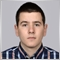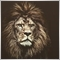# Indicator prev calculated variable issue6589

hi,

I am experiencing problems with the prev calculated variable in indicators for mt4. In the first calculation its returning 10000(for example), in the next one - 0 (so far its normal).But after that it starts looping - one time 0, one time the same 10000, and this is causing the indicator to malfunction.

This is the code, nothing extraordinary :

```   int limit=(prev_calculated==0)?(rates_total-3):(rates_total-prev_calculated);
for(int i=limit;i>=0;i--)
```

Thank you38676

Stanislav Ivanov:

hi,

I am experiencing problems with the prev calculated variable in indicators for mt4. In the first calculation its returning 10000(for example), in the next one - 0 (so far its normal).But after that it starts looping - one time 0, one time the same 10000, and this is causing the indicator to malfunction.

This is the code, nothing extraordinary :

Thank you

Provide a complete test case to reproduce the issue if you want help.6589

I am using a renko offline chart to calculate some values, and using the code you saw to make a look to process data and go through the renko bars. The issue is that every other OnCalculate event the prev_counted returns 0, although it is not 0.38676

Stanislav Ivanov:
I am using a renko offline chart to calculate some values, and using the code you saw to make a look to process data and go through the renko bars. The issue is that every other OnCalculate event the prev_counted returns 0, although it is not 0.

You should provide all information from the start.

That's most probably due to the Renko generator.627

another formula to set this limit is :

```int limit = MathMax(rates_total - prev_calculated, 2);
for (int i=0; i<limit; i++){
//....
}```9829

what do you return in the OnCalculate() function?